Cylinder Surface Normals and Integrands

The following is a parametric representation of a cylinder surface that is coaxial with the z-axis (the cylinder ends will be included later)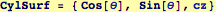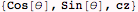The infinitessimal surface vectors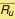and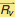for the cylinder surface are obtained by differentiation; they will be used to find the surface patch d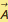.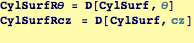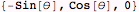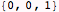The surface normal given by×for the cylinder surface, there for the following (multiplied by dθ dz) is the infinitessimal oriented surface patch d.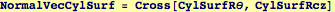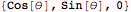The integrand to be evaluated over the cylinder surface is the vector potential, dotted into the normal vector.  Because of the cylindrical symmetry of this model, we can convert to cylindrical coordinates.  One set of coordinates is for the cylinder surface (ξ → R Cos[θ], η → R Sin[θ]) for fixed radius R (which is a model parameter) and another set of coordinates for where we will be testing the potential (x → ρ Cos[α], y → ρ Sin[α]).  Because the potential must be independent of α, we might as well set it to zero.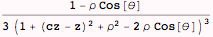We have a choice whether to integrate over cz ∈  (-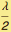,)  or  θ ∈ (0, 2π) first.  If we can a closed form for the cylinder surface over cz and then the cylinder end over r, then we can integrate the sum of these over θ together.

Try and see if we can do one of the two integrals---we have a choice of integrating over θ or (ζ for the cylinder sides, and  R) for the cylinder ends. We find a closed form for integrating  ζ for the sides and R for the top, and then subsequently numerically integrate θ for (0,2 π).

This will take a while to finish, even on a very fast  machine (as of 2007, one minute to do the integral and about 6 hours minutes to simplify the expression).
For this reason, I' ve set up an option to read the result over the web, instead of having each of your computers spend time on a previously computed result:
(NOTE: As of Mathematica 6.01 Oct. 2007,  If we first try the definite integral over θ ∈ (0, 2 π) , we get  ZERO which is  an incorrect result (I will include a discussion of why it is incorrect in the future))

 Created by Wolfram Mathematica 6.0  (23 October 2007)